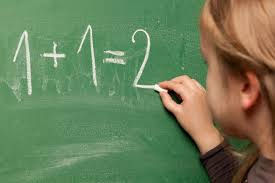top of page

# Math-U-See

"Math-U-See is designed to teach students specific skills that build as the student progresses. This systematic and cumulative approach uses a definite, logical sequence of concept instruction. The Math-U-See system is structured with step-by-step procedures for introducing, reviewing, practicing, and mastering concepts. Each lesson teaches using multi-sensory tools such as videos, manipulatives, and other resources, designed to appeal to any type of learner."

Please take a minute to look at their website:

https://www.mathusee.com/parents/why-math-u-see/Common signs of dyscalculia include trouble:

• Grasping the meaning of quantities or concepts like biggest vs. smallest

• Understanding that the numeral 5 is the same as the word five, and that these both mean five items

• Remembering math facts in school, like times tables

• Counting money or making change

• Estimating time

• Judging speed or distance

• Understanding the logic behind math

• Holding numbers in their head while solving problems

Understood.org

If your child struggles in math, it may be because of DYSCALCULIA

Dyscalculia, is a math learning disability that impairs an individual’s ability to represent and process numerical magnitude in a typical way. Dyscalculia is sometimes called “number dyslexia” or “math dyslexia.” Common symptoms of dyscalculia include difficulty with number sense, fact and calculation, and mathematical reasoning. This may present as difficulty linking numbers and symbols to amounts and directions, making sense of money, or telling time on an analog clock. Roughly 5%-7% of students in the United States have this math-based learning disability.